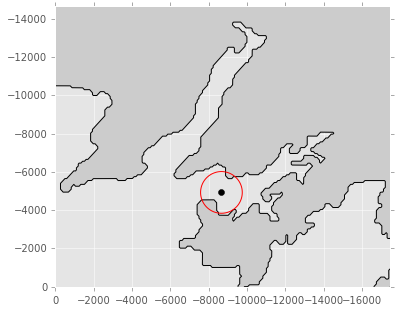# Convert to and from UTM coordinates with pyproj

This post is a quick note on how to convert to and from UTM coordinates using pyproj.

As the end of the year approaches my TODO list gets bigger and bigger and my posts get smaller and smaller... Well, this one is small but I find it useful. I always need to check how to do this every I need to convert to/from UTM coordinates.

pyproj is used under the hood of several python packages like basemap, cartopy, pyresample. So, if you have any of one those above installed, you probably already have pyproj as well.

In :
from pyproj import Proj


Here is am example from real life case that I had to deal recently. Someone gave me the corners of a map in UTM but the sampling site positions as lon, lat collected in WGS84. Here are the data:

In :
import numpy as np
from pandas import DataFrame

core = (-44.6360, -23.2278)

print('\nSampling site lon %s, lat %s\n' % (core, core))

x = (529025.00, 529114.00, 545227.00, 545582.00)
y = (7422210.00, 7422343.00, 7435702.00, 7435741.00)

df = DataFrame(np.c_[x, y], columns=['Meters East', 'Meters South'])
df


Sampling site lon -44.636, lat -23.2278


Out:
Meters East Meters South
0 529025 7422210
1 529114 7422343
2 545227 7435702
3 545582 7435741

All that is needed to solve that problem is a pyproj object for Zone 23K (near Paraty-Brazil) using Datum WGS-84. Note that we need to specify that we are in the South Hemisphere.

In :
myProj = Proj("+proj=utm +zone=23K, +south +ellps=WGS84 +datum=WGS84 +units=m +no_defs")


The next cell uses the object created (myProj) to convert the UTM positions to longitude and latitude. That is why we need the inverse keyword.

In :
lon, lat = myProj(df['Meters East'].values, df['Meters South'].values, inverse=True)


To convert back just from comparison:

In :
UTMx, UTMy = myProj(lon, lat)


And now a quick table to show the results in the notebook,

In :
DataFrame(np.c_[UTMx, UTMy, lon, lat], columns=['UTMx', 'UTMy', 'Lon', 'Lat'])

Out:
UTMx UTMy Lon Lat
0 529025 7422210 -44.716128 -23.309315
1 529114 7422343 -44.715260 -23.308112
2 545227 7435702 -44.558073 -23.187077
3 545582 7435741 -44.554606 -23.186714

Now I can make a map to show the sampling site.

In :
import matplotlib.pyplot as plt
from mpl_toolkits.basemap import pyproj
from mpl_toolkits.basemap import Basemap

fig, ax = plt.subplots(figsize=(6, 6))
m = Basemap(projection='merc',
urcrnrlat=lat, llcrnrlat=lat,
urcrnrlon=lon, llcrnrlon=lon,
resolution='f',
suppress_ticks=False,
ax=ax)
m.fillcontinents()
m.drawcoastlines()
m.ax = ax
pt = m.plot(core, core, 'ko', latlon=True)

lons, lats, xs, ys = m.makegrid(200, 200, returnxy=True)

gc = pyproj.Geod(a=m.rmajor, b=m.rminor)

distances = np.zeros(lons.size)

for k, (lo, la) in enumerate(zip(lons.flatten(), lats.flatten())):
_, _, distances[k] = gc.inv(core, core, lo, la)

distances = distances.reshape(200, 200)  # In km.

# Plot perimeters of equal distance.
levels =   # [50, 100, 150]
cs = m.contour(xs, ys, distances, levels, colors='r')

WARNING: x coordinate not montonically increasing - contour plot
may not be what you expect.  If it looks odd, your can either
adjust the map projection region to be consistent with your data, or
(if your data is on a global lat/lon grid) use the shiftdata
method to adjust the data to be consistent with the map projection
region (see examples/shiftdata.py).In :
HTML(html)

Out:

This post was written as an IPython notebook. It is available for download or as a static html.